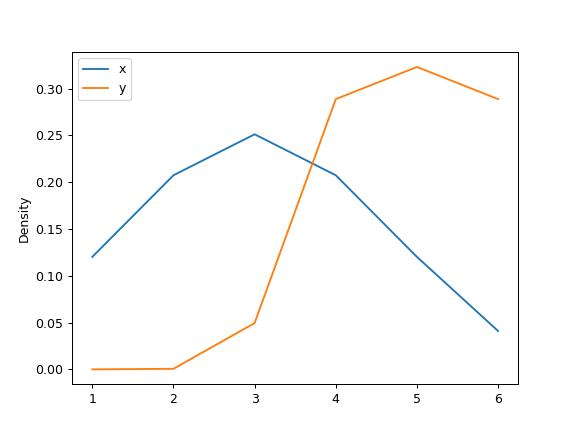# pandas.Series.plot.density¶

`Series.plot.``density`(bw_method=None, ind=None, **kwargs)[source]

Generate Kernel Density Estimate plot using Gaussian kernels.

In statistics, kernel density estimation (KDE) is a non-parametric way to estimate the probability density function (PDF) of a random variable. This function uses Gaussian kernels and includes automatic bandwidth determination.

Parameters
bw_methodstr, scalar or callable, optional

The method used to calculate the estimator bandwidth. This can be ‘scott’, ‘silverman’, a scalar constant or a callable. If None (default), ‘scott’ is used. See `scipy.stats.gaussian_kde` for more information.

indNumPy array or int, optional

Evaluation points for the estimated PDF. If None (default), 1000 equally spaced points are used. If ind is a NumPy array, the KDE is evaluated at the points passed. If ind is an integer, ind number of equally spaced points are used.

**kwargs

Additional keyword arguments are documented in `pandas.%(this-datatype)s.plot()`.

Returns
matplotlib.axes.Axes or numpy.ndarray of them

`scipy.stats.gaussian_kde`

Representation of a kernel-density estimate using Gaussian kernels. This is the function used internally to estimate the PDF.

Examples

Given a Series of points randomly sampled from an unknown distribution, estimate its PDF using KDE with automatic bandwidth determination and plot the results, evaluating them at 1000 equally spaced points (default):

```>>> s = pd.Series([1, 2, 2.5, 3, 3.5, 4, 5])
>>> ax = s.plot.kde()
```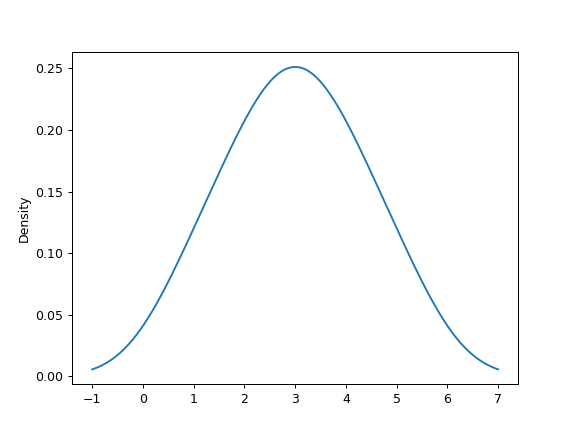A scalar bandwidth can be specified. Using a small bandwidth value can lead to over-fitting, while using a large bandwidth value may result in under-fitting:

```>>> ax = s.plot.kde(bw_method=0.3)
```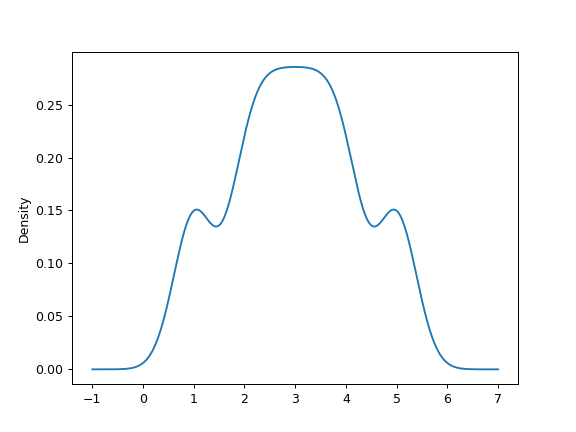```>>> ax = s.plot.kde(bw_method=3)
```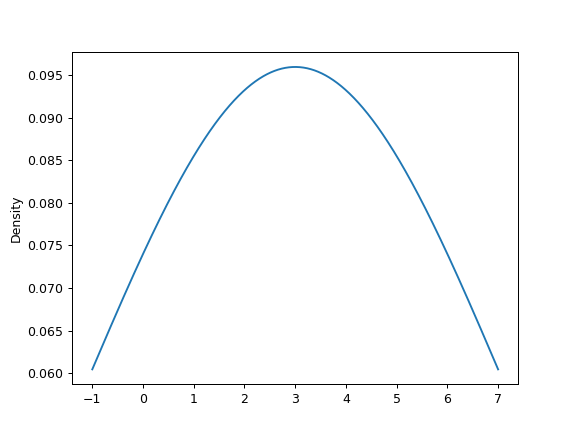Finally, the ind parameter determines the evaluation points for the plot of the estimated PDF:

```>>> ax = s.plot.kde(ind=[1, 2, 3, 4, 5])
```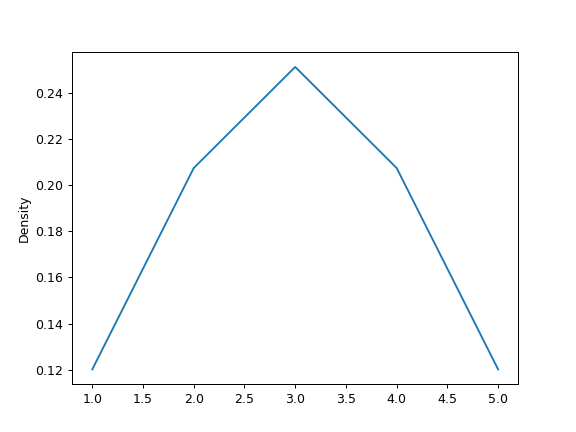For DataFrame, it works in the same way:

```>>> df = pd.DataFrame({
...     'x': [1, 2, 2.5, 3, 3.5, 4, 5],
...     'y': [4, 4, 4.5, 5, 5.5, 6, 6],
... })
>>> ax = df.plot.kde()
```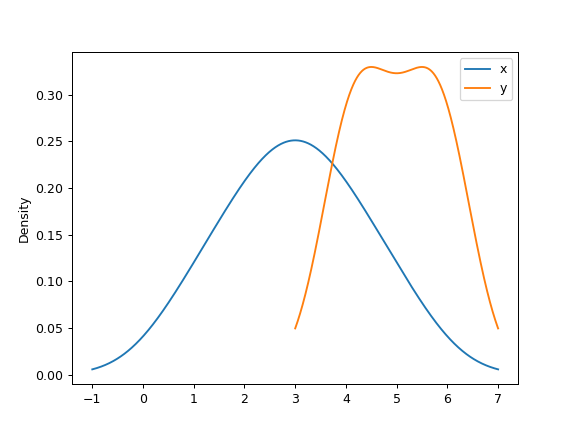A scalar bandwidth can be specified. Using a small bandwidth value can lead to over-fitting, while using a large bandwidth value may result in under-fitting:

```>>> ax = df.plot.kde(bw_method=0.3)
```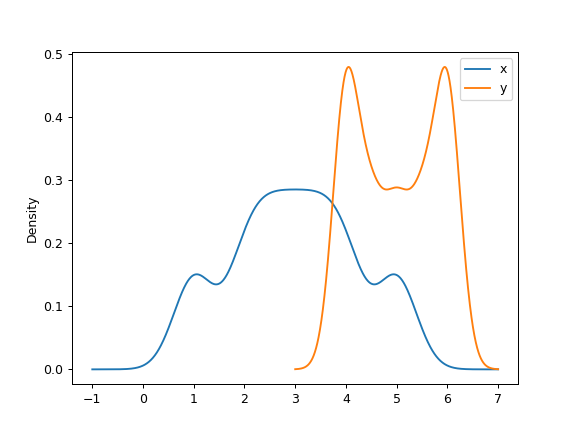```>>> ax = df.plot.kde(bw_method=3)
```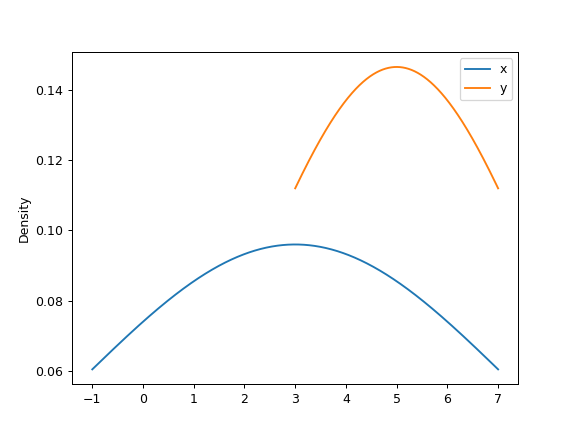Finally, the ind parameter determines the evaluation points for the plot of the estimated PDF:

```>>> ax = df.plot.kde(ind=[1, 2, 3, 4, 5, 6])
```